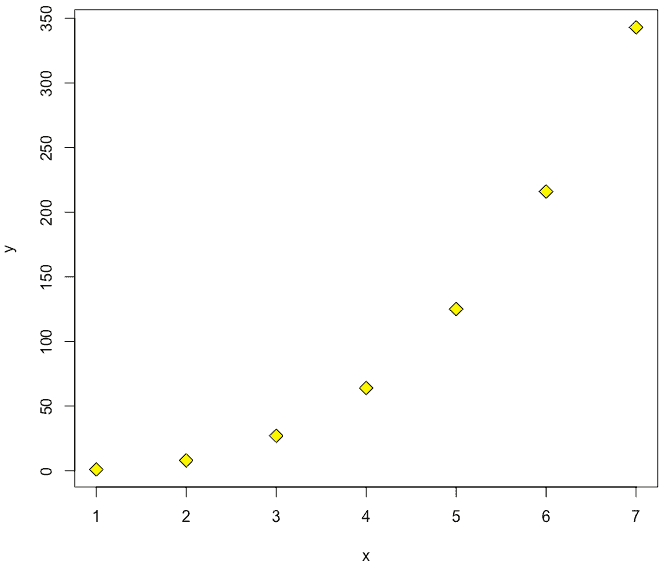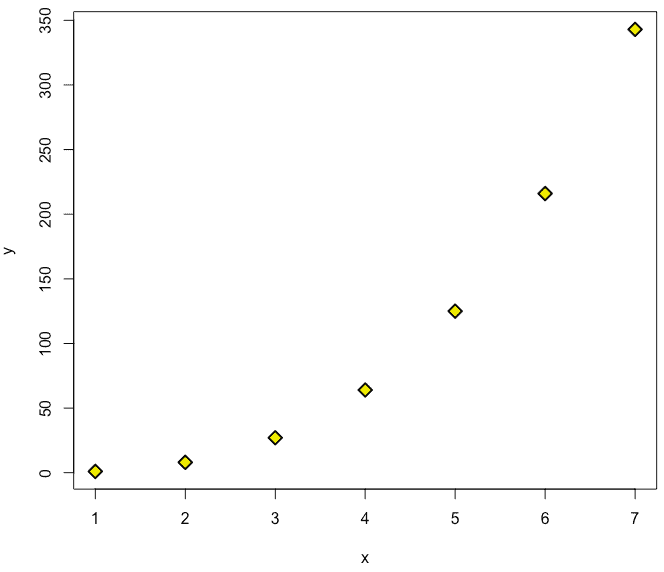# lwd in R: How to Define Line Width While Creating a Plot

The lwd is one of the graphical parameters of the plot() function. To create a chart from a dataset in R, use the plot() function. In R base plot functions, the option lty is used to define a line type, and lwd is used to define a line width.

## lwd in R

The lwd in R stands for line width. While creating a plot in R, the lwd defines the width of a line. It is a line width relative to the default (default=1). If you pass lwd = 2, then it is twice as wide.

The syntax of the plot() function is following.

``plot( x, y, pch = 1, cex = 1, col = 1, bg = 0, lwd = 1, lty = 1, type = "p", add = FALSE )``

As you can see that one of the arguments is lwd, and its value is 1.

Let’s create a plot based on the equation: y = x ^ 3.

``````x <- c(1, 2, 3, 4, 5, 6, 7)
y <- c(1, 8, 27, 64, 125, 216, 343)

plot(x, y, pch=23, col = "black", bg = "yellow", lwd=1, cex=1.5)``````

#### OutputIn this plot, we have passed lwd = 1 value, and you can see that the diamond point’s border has width 1.

If we change the lwd = 2, then the width will be increased.Now, you can clearly see the width of the diamond point, and it is clearly visible. So, this is where the lwd parameter is useful while plotting your dataset into a graph.

You can customize many features of your graphs using the plot() function. For example, you can modify the colors, axes, titles, and fonts using various graphic options.

To specify all these options, use the par( ) function. If you set parameter values here, the changes will affect the rest of the session or until you change them again. The format is par(optionname=value, optionname=value, ).

Use the pch option to define the symbols for your plotting points. From symbols 21 through 25, you can define the border color (col=) and fill color (bg=). There are various options that can help you to specify the color for various properties of the plot.

## Conclusion

For plotting points in R, use the lty and lwd options for changing lines type and thickness in R.

That is it for lwd in R tutorial.